# Excel Multiplication Operator

## Multiplication Operator

Multiplication uses the `*` symbol in Excel.

How to multiply cells:

1. Select a cell and type (`=`)
2. Select a cell
3. Type (`*`)
4. Select another cell
5. Hit enter

You can add more cells to formula by typing (*) between the cells.

Let's have a look at some examples.

## Multiplying Manual Entries

Step by step:

1. Type `A1(=)`
2. Type `2*2`
3. Hit enter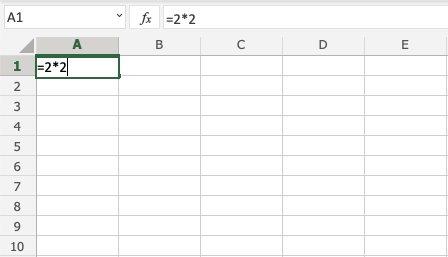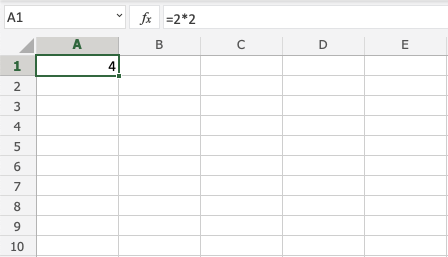## Multiplying Two Cells

Let's add some numbers to work with. Type the following values: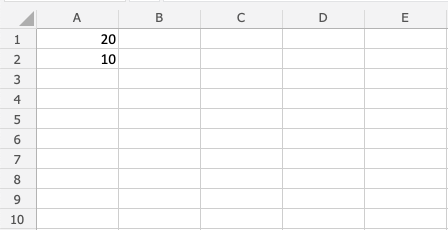Step by step:

1. Type `A3(=)`
2. Select `A1`
3. Type (`*`)
4. Select `A2`
5. Hit enter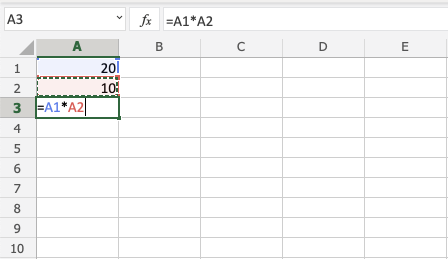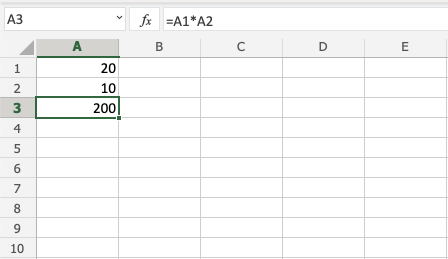## Multiplying Using Absolute Reference

You can lock a cell and multiply it with other cells.

How to do it, step by step:

1. Select a cell and type (`=`)
2. Select the cell you want to lock and add two dollar signs (`\$`) before the column and row
3. Type (`*`)
4. Select another cell
5. Hit enter
6. Fill the range

Let's have a look at an example where we multiply `B(5)` with the range `A1:A10` using absolute reference and the fill function.

Type the values: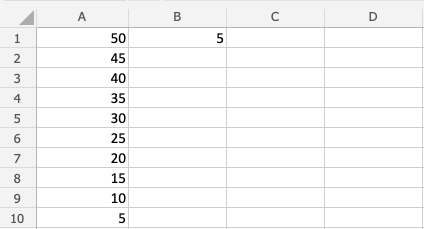Step by step:

1. Type `C1(=)`
2. Select `B1` type dollar sign before column and row `\$B\$1`
3. Type (`*`)
4. Select `A1`
5. Hit enter
6. Fill `C1:C10`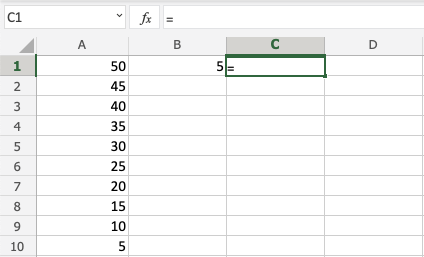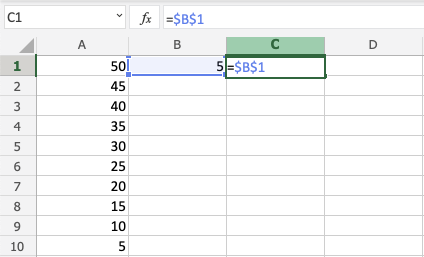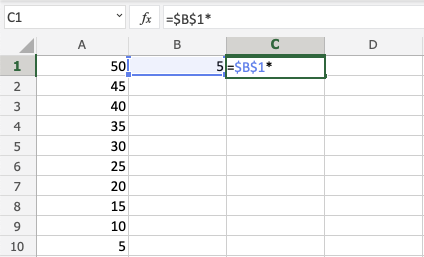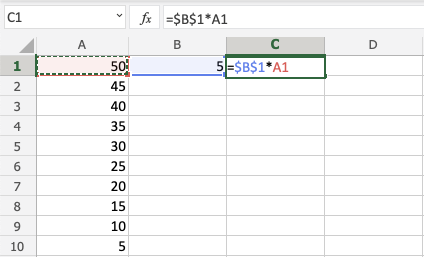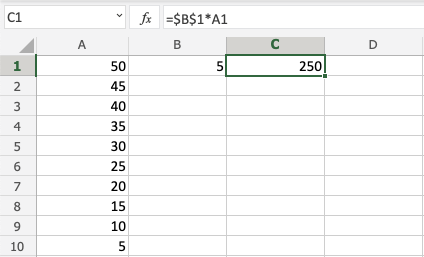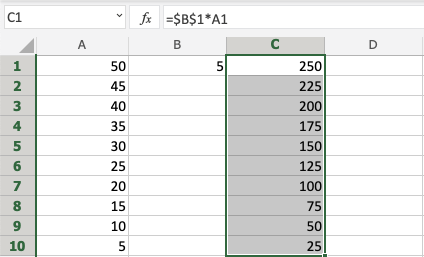You got it! You have successfully used absolute reference to multiply `B1(5)` with the range `A1:A10`.